Skip to main content

# Modeling and Simulation of an Invasive Mild Hypothermic Blood Cooling System

## Abstract

Nowadays, mild hypothermia is widely used in the fields of post-cardiac arrest resuscitation, stroke, cerebral hemorrhage, large-scale cerebral infarction, and craniocerebral injury. In this paper, a locally mixed sub-low temperature device is designed, and the cold and hot water mixing experiment is used to simulate the human blood transfer process. To set a foundation for the optimization of the heat transfer system, the static characteristics are analyzed by building the mathematic model and setting up the experimental station. In addition, the affection of several key structure parameters is researched. Through experimental and simulation studies, it can be concluded that, firstly, the mathematical model proved to be effective. Secondly, the results of simulation experiments show that 14.52 °C refrigeration can reduce the original temperature of 33.42 °C to 32.02 °C, and the temperature of refrigerated blood rises to 18.64 °C, and the average error is about 0.3 °C. Thirdly, as the thermal conductivity of the vascular sheath increases, the efficiency of the heat exchange system also increases significantly. Finally, as the input cold blood flow rate increases, the mass increases and the temperature of the mixed blood temperature decreases. It provides a research basis for subsequent research on local fixed-point sub-low temperature control technology.

## Introduction

Recently, mild therapeutic hypothermia (refers to deliberate reduction of the core body temperature, typically to a range of 32 °C to 34 °C) has been widely used in the treatment of post-cardiac resuscitation, stroke, cerebral hemorrhage, large-area cerebral infarction and craniocerebral injury [1,2,3,4]. A group of patients with severe craniocerebral trauma were treated by body surface cooling method used by Metz in 1996. Seven of the 10 patients recovered to the normal state. In 1996, Reith also found that timely reduction of body temperature after stroke can help reduce mortality by clinical observation of 390 stroke patients [5, 6]. Twelve patients with cardiac arrest and resuscitation were rescued through mild hypothermia at the First Affiliated Hospital of Wenzhou Medical University from 2013 to 2017, and nine patients survived. Induced hypothermia is an acknowledged useful therapy for treating conditions that leads to cell and tissue damage caused by ischemia, including traumatic brain injury, stroke, and cardiac arrest [7,8,9].

Mild therapeutic hypothermia mainly uses drug cooling and physical cooling. The method of cooling the drug is to induce the cooling of the central nervous system by intravenously injecting drugs such as dihydrocapsaicin, vasopressin, opioids and endocannabinoids into the central nervous system [10,11,12]. Drug-induced hypothermia has the characteristics of low temperature control, low incidence of chills and neuroprotection. However, low temperature affects the metabolism of drugs and increases the toxicity of drugs. At present, the dose and adverse reactions of drug-lowering drugs still need to be further clarified [13, 14]. Therefore, the hyaluronic treatment method currently used in clinical practice is still the treatment of physical cooling and physical combined drug cooling. Physical cooling includes body surface cooling and intravascular heat exchange. Body surface cooling uses ice blankets, alcohol rubbing baths, body parts of the large blood vessels (such as the groin, armpits and neck) coated with ice packs, circulating cold air treatment and / or rectal or bladder ice water rinse to induce and maintain body temperature; there are also methods that use the head to focus on sub-hypothermia, such as ice caps or semiconductor-applied cooling [15,16,17]. Although the body surface cooling method can reach the target temperature, the induction process of this type of cooling method is long and the temperature fluctuation range is large. The experimental study found that the time to reach the sub-low temperature through the body surface cooling is 7.0 ± 2.6 h, and the rewarming time is 8.2 ± 0.8 h, at the same time, the time to reach sub-low temperature by intravascular heat exchange was only 4.8 ± 1.9 h, and the rewarming time was 6.0 ± 0.5 h. Intravascular heat exchange cooling is the use of an interventional method to insert a temperature control catheter into the human arterial blood vessel, directly cooling/ rewarming the blood, and the cooling saline is pumped into the catheter to fully contact the blood for heat exchange, and then flow back through the catheter to the temperature control. In the system [18, 19], however, because it belongs to systemic cooling, it will bring some complications, the incidence of hypotension, hypovolemia, bradycardia, and arrhythmia is 36% to 58% . The incidence of complications in the digestive system and metabolic system is 95.5% , and the diaphragm is induced by hypothermia . At the same time, insulin secretion is inhibited during cooling, and hyperglycemia is prone to occur [20, 23,24,25].

Many domestic and foreign scholars have conducted many animal experiments. The results show that selective brain hypothermia can also significantly reduce secondary brain damage and brain edema in cerebral ischemia and experimental brain injury animals, which can reduce harmful substances and protect nerve function and improve survival rate [26,27,28,29]. Therefore, there is a need in the clinic for a treatment technique that can locally achieve and maintain a sub-hypotherm state to improve the effect of hypothermia treatment and reduce the complications caused by systemic cooling . In this paper, a local sub-hypotherm device that can achieve mixed cooling is designed to provide a research basis for the subsequent study of local fixed-point sub-hypothermia control technology.

## Invasive Mild Hypothermic Blood Cooling System

The blood cooling process is shown in Figure 1. Firstly, the blood in the body is pumped to the external temperature control device by the blood pump and the blood vessel sheath. In addition, the temperature control device cools the pumped blood. Finally, the cooled blood is introduced into the blood vessel in the tissue to be cooled through the vascular sheath by the blood pump.Figure 1

The heat exchange process is divided into three stages. The first stage is the process of pumping the blood from the vascular sheath to the tissue to be cooled, which is the partition wall heat exchange; the second stage is the cooling of the blood at the vascular sheath outlet. In the arterial hot blood mixing process, this stage is mixed heat exchange; the third stage is the heat exchange between the mixed blood and the tissue.

At present, the common vascular sheath insertion sites are the femoral artery and the carotid artery. In the first stage of the wall heat exchange process, since the blood flow direction in the artery is from the heart end to the body tissues, when the vascular sheath is inserted by the femoral artery, it is the countercurrent wall heat exchange. It is a downstream wall heat exchange, when the sheath is inserted by the carotid artery.

## Mathematic Formulation

In order to study the temperature change in blood vessels, the complex shape and distribution of blood vessels are first simplified, and the heat transfer between the blood vessels and the cold blood in the blood vessel sheath is discussed. With hypothesis below:

1. 1)

Ignore the influence of blood flow pulsation;

2. 2)

Ignore the influence of blood vessel wall;

3. 3)

Ignore the tissue influence of hot and cold blood heat exchange.

### Concurrent Heat Transfer

When the vascular sheath is inserted into the blood vessel at the upper end of the heart, the cold blood in the vascular sheath flows in the same direction as the blood in the blood vessel, and belongs to the downstream heat exchange (Figure 2). The heat transfer is divided into two stages. The first stage is the exchange of heat between the hot and cold liquid through the vascular sheath, and the second stage is the mixed heat exchange between the hot and cold liquid at the outlet of the vascular sheath.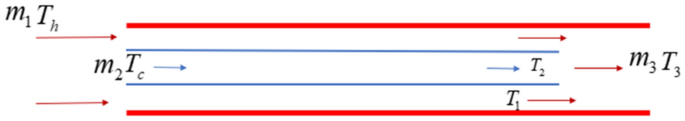Figure 2

#### The First Stage

Selecting a small segment of the x-position of the vascular sheath for energy analysis, the heat lost by the hot blood is just the heat gained by the cold blood

$$- \dot{m}_{1} C_{p1} {\text{d}}T_{h} = {\text{d}}Q = \dot{m}_{2} C_{p2} {\text{d}}T_{c} ,$$
(1)

where Cp1 is the heat capacity flow rate of hot blood, and temperature is Th; Cp2 is the heat capacity flow rate of hot blood, and temperature is Tc; Q is heat transfer.

Assuming the heat exchange area of the hot and cold fluid is dA at this time, and the heat transfer amount of the micro-element is obtained by Newton’s law of cooling:

$${\text{d}}Q = U\Delta T{\text{d}}A,$$
(2)

where U is the heat transfer coefficient. And

$${\text{d}}\Delta T = {\text{d}}T_{h} - {\text{d}}T_{c} .$$
(3)

Bring Eq. (1) into Eq. (3) to get:

$${\text{d}}\Delta T = - {\text{d}}Q\left( {\frac{1}{{\dot{m}_{1} C_{p1} }} + \frac{1}{{\dot{m}_{2} C_{p2} }}} \right).$$
(4)

Substitute Eq. (2) into Eq. (4) and sort out the equilibrium of the microelement:

$$\frac{{{\text{d}}(\Delta T)}}{\Delta T} = - U\left( {\frac{1}{{\dot{m}_{1} C_{p1} }} + \frac{1}{{\dot{m}_{2} C_{p2} }}} \right){\text{d}}A.$$
(5)

Integrate Eq. (5) the boundary condition:

$$A = 0 ,\;\Delta T = T_{h} - T_{c} .$$

Solve:

$$\Delta T = \left( {T_{A} - T_{c} } \right)e^{{ - U\left( {\frac{1}{{\dot{m}_{1} C_{p1} }} + \frac{1}{{\dot{m}_{2} C_{p2} }}} \right)A}} .$$
(6)

Combine Eq. (6) and Eq. (2):

$${\text{d}}Q = U\left( {T_{h} - T_{c} } \right)e^{{ - U\left( {\frac{1}{{\dot{m}C_{p1} }} + \frac{1}{{\dot{m}C_{p2} }}} \right)A}} {\text{d}}A.$$
(7)

When $$A = 0, \, Q = 0,$$ the integral of Eq. (7) is:

$$Q = \frac{{T_{h} - T_{c} }}{{\frac{1}{{\dot{m}_{1} C_{{p_{1} }} }} + \frac{1}{{\dot{m}_{2} C_{{p_{2} }} }}}}\left( {1 - e^{{ - U\left( {\frac{1}{{\dot{m}_{1} C_{{p_{1} }} }} + \frac{1}{{\dot{m}_{2} C_{{p_{2} }} }}} \right)A}} } \right).$$
(8)

When A = 0,

$$T_{1} = T_{h} - \frac{Q}{{\dot{m}_{1} C_{p1} }},$$
(9)
$$T_{2} = T_{c} + \frac{Q}{{\dot{m}_{2} C_{p2} }}.$$
(10)

Combining Eqs. (8)‒(10),

$$T_{1} = T_{h} - \frac{{T_{\hbar } - T_{c} }}{{1 + \frac{{\dot{m}_{ 1} C_{p1} }}{{\dot{m}_{ 2} C_{p2} }}}}\left( {1 - e^{{ - U\left( {\frac{1}{{\dot{m}_{ 1} C_{p1} }} + \frac{1}{{\dot{m}_{ 2} C_{p2} }}} \right)A}} } \right),$$
(11)
$$T_{1} = T_{c} { + }\frac{{T_{\hbar } - T_{c} }}{{1 + \frac{{\dot{m}_{ 2} C_{p 2} }}{{\dot{m}_{ 1} C_{p 1} }}}}\left( {1 - e^{{ - U\left( {\frac{1}{{\dot{m}_{ 1} C_{p1} }} + \frac{1}{{\dot{m}_{ 2} C_{p2} }}} \right)A}} } \right).$$
(12)

#### The Second Stage

Ignore the loss of other heat, according to energy quality and energy conservation:

$$\dot{m}_{3} = \dot{m}_{1} + \dot{m}_{2} ,$$
(13)
$$\dot{m}_{3} C_{p3} T_{3} = \dot{m}_{1} C_{p1} T_{1} + \dot{m}_{2} C_{p2} T_{2} ,$$
(14)
$$T_{3} = \frac{{C_{1} A_{1} T_{1} + C_{2} A_{2} T_{2} }}{{C_{1} A_{1} + C_{2} A_{2} }}.$$
(15)

### Countercurrent Heat Transfer

When the vascular sheath is inserted into the blood vessel at the upper end of the heart, the cold blood in the vascular sheath is opposite to the blood flow in the blood vessel and belongs to countercurrent heat exchange (Figure 3). The heat transfer is divided into two stages. The first stage is the exchange of heat between the hot and cold liquid through the vascular sheath, and the second stage is the mixed heat exchange between the hot and cold liquid at the outlet of the vascular sheath.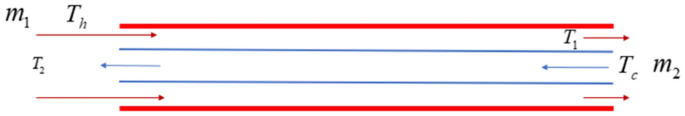Figure 3

According to conservation of energy,

$$- \dot{m}_{1} C_{p1} dT_{{\hat{h}}} = {\text{d}}Q = - \dot{m}_{2} C_{p2} {\text{d}}T_{c} .$$
(16)

Newton’s law of cooling:

$${\text{d}}Q = U\Delta T{\text{d}}A,$$
(17)
$$T_{1} = T_{h} - \frac{{T_{A} - T_{c} }}{{1 - \frac{{\dot{m}_{1} C_{p1} }}{{\dot{m}_{2} C_{p2} }}}}\left( {1 - e^{{U\left( {\frac{1}{{\dot{m}_{2} C_{p2} }} - \frac{1}{{\dot{m}_{1} C_{p1} }}} \right)A}} } \right),$$
(18)
$$T_{2} = T_{c} - \frac{{T_{h} - T_{c} }}{{\frac{{\dot{m}_{2} C_{p2} }}{{\dot{m}_{1} C_{p1} }} - 1}}\left( {1 - e^{{U\left( {\frac{1}{{\dot{m}_{2} C_{p2} }} - \frac{1}{{\dot{m}_{1} C_{{p_{1} }} }}} \right)A}} } \right).$$
(19)

### Calculation of heat transfer coefficient

The heat transfer coefficient U is

$$U = \frac{1}{{\frac{1}{{h_{\text{hot}} }} + \frac{1}{{h_{\text{cool}} }} + \frac{\delta }{\lambda }}}.$$
(20)

Among them, $$h_{\text{hot}}$$ and $$h_{\text{cool}}$$ is the surface heat exchange coefficient of hot and cold liquid, $$\delta$$ is wall thickness, $$\lambda$$ is thermal conductivity of the tube wall,

$$h{ = }Nu_{f} \frac{\lambda }{D},$$
(21)

where D is the diameter of the runner, and

$$Re = \frac{PvD}{\mu },$$
(22)

where Re is Reynolds number. v, ρ, μ are the fluid velocity, density and viscosity coefficient, respectively.

When $$Re \le 2200$$, using the Seider-Tate formula

$$Nu_{f} = 1.86Re_{f}^{1/3} Pr_{f}^{1/3} \left( {\frac{{d_{e} }}{L}} \right)^{1/3} \left( {\frac{{\mu_{f} }}{{\mu_{c} }}} \right)^{0.14} .$$
(23)

When $$2200 \le Re \le 10000,$$ using the Gnilinski formula

$$Nu_{f} = \frac{{(f/8)(Re - 1000)Pr_{f} }}{{I + 12.7\sqrt {f/8} \left( {Pr_{f}^{2/3} - 1} \right)}}\left[ {I + \left( {\frac{d}{l}} \right)} \right]\left( {\frac{{Pr_{f} }}{{Pr_{w} }}} \right)^{0.11} ,$$
(24)
$$f = (1.82\lg Re - 1.64)^{ - 2} .$$
(25)

When $$Re > 10000,$$

$$Nu_{f} = 0.023Re_{f}^{0.8} Pr_{f}^{n} .$$
(26)

## Comparison of Results from Simulation and Experiments

In order to verify the reliability of the mathematical model, the simulated blood cooling scheme was designed as follows.

1. 1)

Water simulates human blood.

2. 2)

Diaphragm pump simulates heart pumping and the flow rate is set to 500 mL/min.

3. 3)

PU tube with a diameter of 6 mm simulates human arterial vessels.

4. 4)

T-type tee simulates vascular sheath insertion into blood vessels.

5. 5)

Use T-type tee to measure the temperature of the inlet/outlet liquid of hot and cold liquid.

6. 6)

Connection between different caliber pipes through reducer joints.

By varying the diameter, material and length of the vascular sheath, the inlet temperature of the cooling liquid and the inlet flow, the resulting hot and cold liquid outlet temperature is compared to the theoretical model.

The simulation scheme is shown in Figures 4 and 5. The experimental station of the simulated heat transfer system is shown in Figure 6. The water in the refrigerating device and the incubator is pumped separately by the water pump and the temperature of the cold water and the hot water is measured by the sensor. Then, the vascular sheath is inserted into the blood vessel by using a tee. The vascular sheath is also introduced into the blood vessel by using a tee, and the temperature of the hot and cold water after the first-stage heat exchange is measured by a sensor.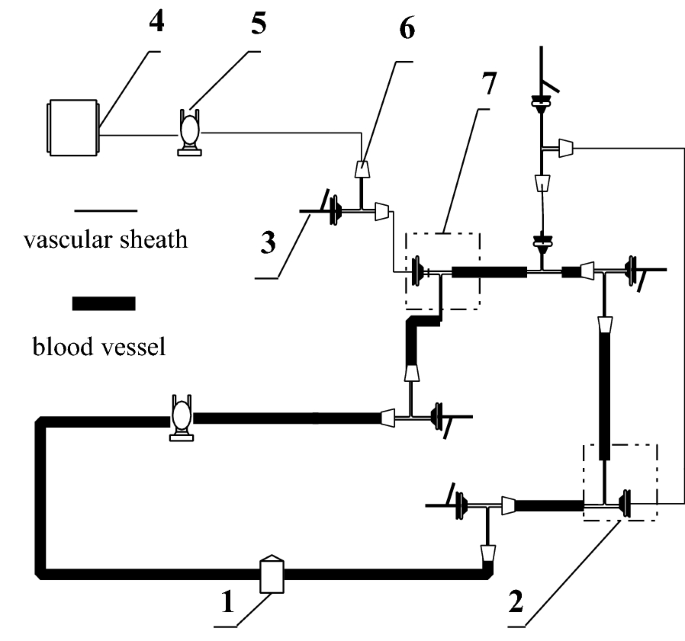Figure 4Figure 5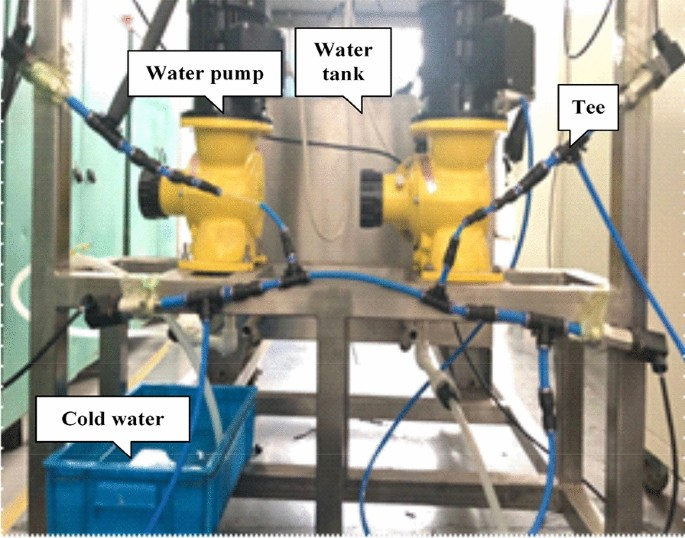Figure 6

The experimental parameters of the first group are shown in Table 1. The measured cold and hot liquid outlet temperature of the countercurrent is compared with the calculated liquid outlet temperature curve of the model, and the model is verified. The comparison results are shown in Table 2, in the countercurrent hot liquid outlet. In the prediction of temperature, the relative error is within 0.014%, and the relative error in the prediction of the countercurrent cold liquid outlet temperature is within 4.7%.

The experimental parameters of the second group are shown in Table 3. The measured cold and hot liquid outlet temperature of the countercurrent is compared with the calculated liquid outlet temperature curve of the model, and the model is verified. The comparison results are shown in Table 4, in the countercurrent hot liquid outlet. In the prediction of temperature, the relative error is within 0.015%, and the relative error in the prediction of the countercurrent cold liquid outlet temperature is within 3.5%.

The experimental parameters of the second group are shown in Table 5. The measured cold and hot liquid outlet temperature of the downstream is compared with the calculated liquid outlet temperature curve of the model, and the model is verified. The comparison results are shown in Table 6, in the downstream hot liquid outlet. In the prediction of temperature, the relative error is within 0.01%, and the relative error in the prediction of the downstream cold liquid outlet temperature is within 2.7%.

The experimental parameters of the second group are shown in Table 7. The measured cold and hot liquid outlet temperature of the countercurrent is compared with the calculated liquid outlet temperature curve of the model, and the model is verified. The comparison results are shown in Table 8, in the countercurrent hot liquid outlet. In the prediction of temperature, the relative error is within 0.29%, and the relative error in the prediction of the countercurrent cold liquid outlet temperature is within 1.3%.

Figures 7 and 8 compare the measured cold and hot liquid outlet temperature of the forward and reverse flow with the model calculated liquid outlet temperature curve, and the temperature variables of the model are verified. The comparison results are shown in Figures 7 and 8. In the prediction of the outlet temperature of the hot fluid, the relative error is less than 0.6%. In the prediction of the downstream temperature of the downstream liquid, the relative error falls within 11.7%. In the prediction of the countercurrent hot liquid outlet temperature, the relative error falls at 0.43%. Within the prediction of the countercurrent cold liquid outlet temperature, the relative error falls within 7%.Figure 7Figure 8

From the result of the error, the relevant parameters of the vascular sheath are changed, and the theoretical value of the experiment and the model is less wrong. The error may be caused by the measurement error of the temperature sensor and the thermal conductivity of the vascular sheath material, and the error is changed when the flow rate of the cold liquid is changed. It is probably that there is an error in the measurement of the flow rate due to the pulse pump flow of the pulse pump. In general, the theoretical and experimental values have been changing, and the error is within an acceptable range.

## Analysis of Influencing Factors

The physical parameters of blood were brought into the established mathematical model, and the influence of each parameter variable on blood heat transfer was analyzed.

It is obvious in Figures 9, 10, 11, 12, 13 that the outlet temperature changes linearly under the material temperature, length, diameter and cold liquid inlet temperature variable. However, the outlet temperature changes exponentially under the coolant inlet flow variable.Figure 9Figure 10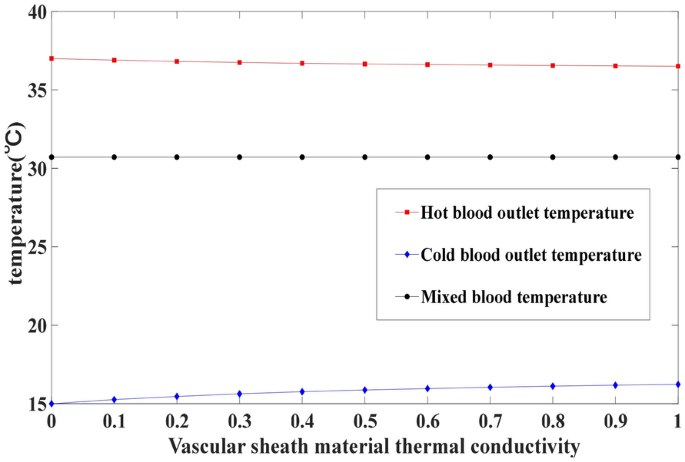Figure 11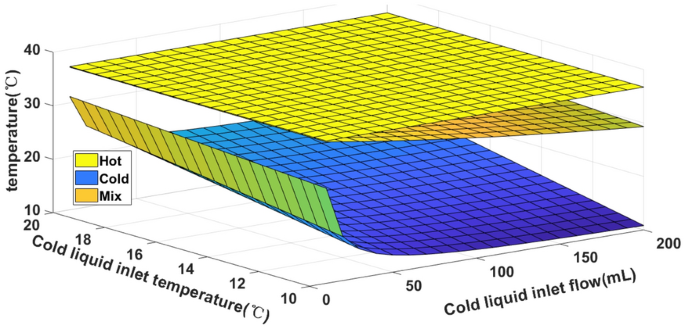Figure 12Figure 13

It can be seen from Figures 9, 10, 11 that in the initial stage of cooling, when the thermal conductivity of the material of the vascular sheath is decreased by 0.1 W/(m·K), the hot blood outlet temperature is reduced by 0.25 °C. When the length of the vascular sheath is shortened from 800 mm to 200 mm, the hot blood outlet temperature is lowered by 0.5 °C. After the thermal conductivity increases, the temperature drop is slowed down. The thermal conductivity of the material is an important factor affecting the heat transfer in the first stage.

After the end of the first stage of heat exchange, the cooling temperature of the hot blood is not obvious, and the cooled blood will have a little temperature rise, indicating that the mixed heat transfer is the main way of blood cooling in the whole heat exchange process.

In the second stage of heat exchange process, the material and diameter of the vascular sheath have no effect on the heat transfer result. For every 1 °C decrease in cold blood, the mixed blood temperature is lowered by 0.3 °C. For every 10 mL of cold blood, the mixed blood temperature is lowered by 0.4 °C.

## Conclusions

In this study, to set a foundation for the follow-up work on local fixed-point hypothermia control technology, a mathematical model of the blood heat transfer was proposed, and its static characteristics are analyzed by researching the key parameters of great influence. The conclusions can be drawn as follows:

1. 1)

The simulation results are consistent well with the experimental results, and the mathematical model in the preceding texts is verified to be highly effective.

2. 2)

Through the blood heat transfer simulation experiment, the results of the live simulation experiment can be obtained. The results of simulation experiments show that 14.52 °C refrigeration can reduce the original temperature of 33.42 °C to 32.02 °C, and the temperature of refrigerated blood rises to 18.64 °C, and the average error is about 0.3 °C. This can be used as a basic reference for the design and optimization of blood cooling devices.

3. 3)

Vascular sheath thermal conductivity is a key parameter in one-stage heat transfer. Higher thermal conductivity materials improve the efficiency of heat exchange.

4. 4)

Cold blood flow and temperature affect the final heat transfer effect. For every 10 mL increase in flow rate, the temperature will decrease by 0.4 °C, and for every one degree Celsius cold blood temperature decrease, the temperature of the mixed blood will decrease by 0.3 °C. Therefore, in practical applications, a suitable range of blood temperature and flow should be found to achieve the effect of blood cooling.

## References

1. 

Y Zhang, J Liu, Y Zhou. Pilot study on cryogenic heat transfer in biological tissues embedded with large blood vessels. Forsch Ingenieurwes, 2002, 67: 188-197.

2. 

K Piironen, M Tiainen, S Mustanoja, et al. Mild hypothermia after intravenous thrombolysis in patients with acute stroke: A randomized controlled trial. Stroke, 2014, 45: 486-491.

3. 

S Blowers, I Marshall, M Thrippleton, et al. How does blood regulate cerebral temperatures during hypothermia?  Scientific Reports, 2018, 8: 7877.

4. 

L Wu, D Wu, T Yang, et al. Hypothermic neuroprotection against acute ischemic stroke: The 2019 update. Journal of Cerebral Blood Flow & Metabolism, 2020, 40(3): 461-481.

5. 

H Fei, G Cheng, A Cheng. Research status of mild hypothermia treatment of spinal cord injury. China Medical Herald, 2012, 9(9): 11-13. (in Chinese)

6. 

RM Martins, JS Teodoro, E Furtado, et al. Mild hypothermia during the reperfusion phase protects mitochondrial bioenergetics against ischemia-reperfusion injury in an animal model of ex-vivo liver transplantation-an experimental study. International Journal of Medical Sciences, 2019, 16(9):1304-1312.

7. 

Nordberg P, Annoni F, Fabio TS. The impact of intra-arrest hypothermia. Current Opinion in Critical Care, 2020, 26(3): 236-241.

8. 

Fukunaga, Hisanori. The effect of low temperatures on environmental radiation damage in living systems: Does hypothermia show promise for space travel? International Journal of Molecular Sciences, 2020, 21(17): 6349.

9. 

L Perretta, R Reed, G Ross, et al. Is there a role for therapeutic hypothermia administration in term infants with mild neonatal encephalopathy? Journal of Perinatology, 2020, 40(3): 522-529.

10. 

AM Omairi, S Pandey. Targeted hypothermia temperature management. [Updated 2020-09-10]. In: StatPearls [Internet]. Treasure Island (FL): StatPearls Publishing, https://www.ncbi.nlm.nih.gov/books/NBK556124/.

11. 

V A Shah, R G Geocadin. Therapeutic hypothermia in neurocritical care. In: S Nelson, P Nyquist, eds. Current clinical neurology. Humana, Cham, 19 March 2020, https://doi.org/10.1007/978-3-030-36548-6_5.

12. 

H Li, X Tan, Q Xue, J H Zhu, et al. Combined application of hypothermia and medical gases in cerebrovascular diseases. Medical Gas Research, 2019, 8(4): 172-175.

13. 

D Azzopardi, NJ Robertson, A Bainbridge, et al. Moderate hypothermia within 6 h of birth plus inhaled xenon versus moderate hypothermia alone after birth asphyxia (TOBY-Xe): A proof-of-concept, open-label, randomized controlled trial. The Lancet Neurology, 2016, 15(2): 145-153.

14. 

X Liao, Z Zhou, M Zhou, et al. Effects of endovascular and surface cooling on resuscitation in patients with cardiac arrest and a comparison of effectiveness, stability, and safety: A systematic review and a meta-analysis. CritCare, 2020, 24(1): 27.

15. 

D Stanger, V Mihajlovic, J Singer, et al. Effects of targeted temperature management on mortality and neurological outcome: A systematic review and meta-analysis. European Heart Journal: Acute Cardiovascular Care, 2018, 7(5): 467-477.

16. 

E Keller, H G Imhof, S Gasser, et al. Endovascular cooling with heat exchange catheters: a new method to induce and maintain hypothermia. Intensive Care Med, 2003, 29: 939-943.

17. 

H M Coleridge, J C Coleridge, C Kidd . Role of the pulmonary arterial baroreceptors in the effects produced by capsaicin in the dog. Journal of Physiology, 1964, 170(170): 272.

18. 

KR Martin, M Naiman, M Espinoza. Using esophageal temperature management to treat severe heat stroke: A case report. J. Neurosci. Nurs., 2020, 52(1): 9-13.

19. 

J Rosman, M Hentzien, M Dramé, et al. A comparison between intravascular and traditional cooling for inducing and maintaining temperature control in patients following cardiac arrest. Anaesthesia Critical Care & Pain Medicine, 2018, 37(2): 129-134.

20. 

Y Zhu, T Xiang, D Hu, et al. Protective effects of mild hypothermia against hepatic injury in rats with acute liver failure. Annals of Hepatology, 2019, 18(5): 770-776.

21. 

X Zhang, X Zhao, C Zhang, et al. Improvement of neurological function and stress in patients with acute massive cerebral infarction by mild hypothermia: A prospective randomized controlled study. Chinese Critical Care Medicine, 2019, 31(8): 958-961. (in Chinese)

22. 

D Azzopardi, AT Chew, A Deierl, et al. Prospective qualification of early cerebral biomarkers in a randomised trial of treatment with xenon combined with moderate hypothermia after birth asphyxia. EBioMedicine, 2019, 47: 484-491.

23. 

J Caroff, R M King, J E Mitchell, et al. Focal cooling of brain parenchyma in a transient large vessel occlusion model: Proof-of-concept. Journal of NeuroInterventional Surgery, 2020, 12: 209-213.

24. 

S M Seyedsaadat, S F Marasco, D J Daly, et al. Selective brain hypothermia: feasibility and safety study of a novel method in five patients. Perfusion, 2020, 35(2): 96-103.

25. 

Y Lutz, T Meiner, L Krames, et al. Selective brain hypothermia for Ischemic MCA-M1 Stroke: Influence of cerebral arterial circulation in a 3D brain temperature model. IEEE Transactions on Biomedical Engineering, 2021, 68(2): 404-415.

26. 

Y Wang, J H Choi, M A Almekhlafi, et al. A system for continuous Pre- to Post-reperfusion intra-carotid cold infusion for selective brain hypothermia in rodent strokemodels. Translational Stroke Research, 2020, https://doi.org/10.1007/s12975-020-00848-3.

27. 

G Cattaneo, M Schumacher, J Wolfertz, et al. Meckel Combined selective cerebral hypothermia and mechanical artery recanalization in acute ischemic stroke: In vitro study of cooling performance. American Journal of Neuroradiology, 2015, 36(11): 2114-2120.

28. 

T Mattingly, S Lownie. Cold blood perfusion for selective hypothermia in acute ischemic stroke. Brain Circulation, 2019, 5(4): 187-194.

29. 

G Cattaneo, M Schumacher, C Maurer, et al. Meckel endovascular cooling catheter for selective brain hypothermia: An animal feasibility study of cooling performance. American Journal of Neuroradiology, 2016, 37(5): 885-891.

30. 

X Xu, P Tikuisis, G Giesbrecht. A mathematical model for human brain cooling during cold-water near-drowning. Appl. Physiol., 1999, 86: 265-272.

Download references

Not applicable

## Funding

Supported by Open Research Project of the State Key Laboratory of Media Convergence and Communication, Communication University of China (Grant No. SKLMCC2020KF002), Fundamental Research Funds for Central Public Welfare Research Institutes, National Key Research and Development Project (Grant No. 2019YFC0121700) and China Postdoctoral Science Foundation (Grant No. 2019M660392).

## Author information

Authors

### Contributions

YS, SW, MC and MJ was in charge of the whole trial; QL and CH wrote the manuscript; YW assisted with sampling and laboratory analyses. All authors read and approved the final manuscript.

### Authors’ Information

Na Wang is a lecturer with a Ph.D at Beihang University, China. She is specialized at study of ischemic preconditioning brain protection, acute cerebral infarction, arterial thrombolysis and venous sinus thrombosis.

Qinghua Liu, born in 1996, is currently a master candidate at North China University of Technology.

Yan Shi is a professor at School of Automation Science and Electrical Engineering, Beihang University, China. He received his doctoral degree in mechanical engineering from Beihang University, China. His research interests include intelligent medical devices and energy-saving technologies of pneumatic systems.

Shijun Wang works at People’s Hospital of Juye County, Heze 274900, China.

Xianzhi Zhang is a professor at Department of Mechanical Engineering, Kingston University, UK. He is specialized at fluid control, measurement and control system.

Chengwei Han received the B.E degree from School of Astronautics, Harbin Institute of Technology, China. He is currently studying in University of New South Wales in Australia for master degree.

Yixuan Wang is currently postdoctoral fellow at Beihang University, China. His research interests include fuel and power systems of UAV, fluid control, measurement and control system.

Maolin Cai is a professor of School of Automation Science and Electrical Engineering, Beihang University, China. He received his doctor degree from Tokyo Institute of Technology, Japan. His research interests include intelligent medical devices, technology of high efficiency and large scale compressed air energy storage, and so on.

Xunming Ji is the chief physician and associate dean of Neurosurgery at Xuanwu Hospital of Capital Medical University, China. Mainly engaged in early treatment of acute cerebral infarction and neuroprotection research. Focus on the study of ischemic preconditioning brain protection, acute cerebral infarction, arterial thrombolysis and venous sinus thrombosis.

### Corresponding authors

Correspondence to Na Wang or Yan Shi.

## Ethics declarations

### Competing Interests

The authors declare no competing financial interests.

## Rights and permissions

Open Access This article is licensed under a Creative Commons Attribution 4.0 International License, which permits use, sharing, adaptation, distribution and reproduction in any medium or format, as long as you give appropriate credit to the original author(s) and the source, provide a link to the Creative Commons licence, and indicate if changes were made. The images or other third party material in this article are included in the article's Creative Commons licence, unless indicated otherwise in a credit line to the material. If material is not included in the article's Creative Commons licence and your intended use is not permitted by statutory regulation or exceeds the permitted use, you will need to obtain permission directly from the copyright holder. To view a copy of this licence, visit http://creativecommons.org/licenses/by/4.0/.

Reprints and Permissions